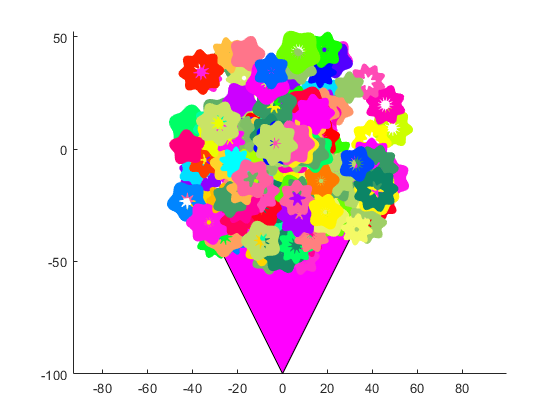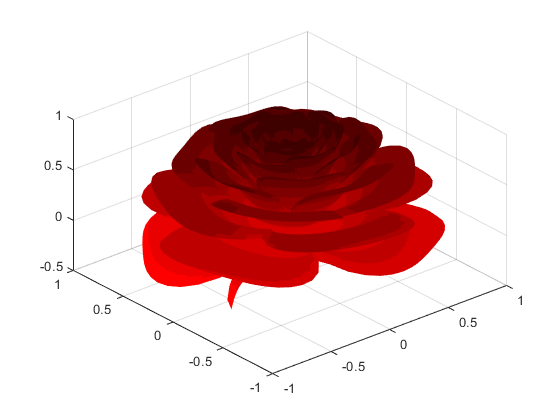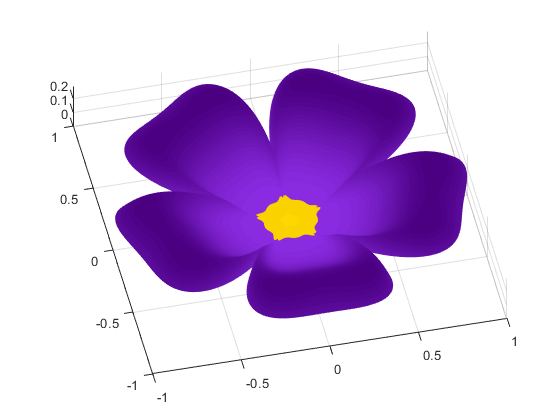Happy Valentines Day!

What to do? It's nearly February 14th, and what, oh what will you get your sweet one? Your very own osculating curve? The one who rounds you up every day? Who's always acute even when you're obtuse?
Don't worry, my friends! The MATLAB Community Blog has you covered. We've been hard at work, combing through the File Exchange, looking for exactly what you need. Because nothing says "I love you" quite like a delicate bouquet of code (and its accompanying image).
There are so many hidden gems on the File Exchange, treasures large and small, patiently waiting to be discovered. Here I present to you Valentine, by Richard Schulman.
ValentineBut wait... there's more! Don't just pique their interest when you can bowl them over.
As an extra floral bonus. I'm going to give you something written by Alison Eele, from our UK office. Alison fashioned this from an algorithm originally written by Paul Nylander (you'll want to check out his good work here: Math Artwork).
The flower algorithm comes from Paul, but this poem is all Alison! I recommend you recite it as you deliver your flowers.
A Valentines Poem by Alison Eele
Roses are [1,0,0]
Violets are [0,0,1]
MATLAB speaks math
(and poetry too!)
Now if that doesn't bring you happiness on Valentines Day, why I don't know what will.
Good luck!
n = 800;
A = 1.995653;
B = 1.27689;
C = 8;
r=linspace(0,1,n);
theta=linspace(-2,20*pi,n);
[R,THETA]=ndgrid(r,theta);
% define the number of petals we want per cycle. Roses have 3 and a bit.
petalNum=3.6;
x = 1 - (1/2)*((5/4)*(1 - mod(petalNum*THETA, 2*pi)/pi).^2 - 1/4).^2;
phi = (pi/2)*exp(-THETA/(C*pi));
y = A*(R.^2).*(B*R - 1).^2.*sin(phi);
R2 = x.*(R.*sin(phi) + y.*cos(phi));
X=R2.*sin(THETA);
Y=R2.*cos(THETA);
Z=x.*(R.*cos(phi)-y.*sin(phi));
% % define a red map for our rose colouring
red_map=linspace(1,0.25,10)';
red_map(:,2)=0;
red_map(:,3)=0;
clf
surf(X,Y,Z,'LineStyle','none')
view([-40.50 42.00])
colormap(red_map)n=800;
r=linspace(0,1,n);
theta=linspace(0,2*pi,n);
[R,THETA]=ndgrid(r,theta);
% define the number of petals we want per cycle. Violets have 5!
petalNum=5;
x = 1 - (1/2)*((5/4)*(1 - mod(petalNum*THETA, 2*pi)/pi).^2 - 1/4).^2;
% update the formula for phi to stop the spiralling effect
phi = (pi/2)*exp(-2*pi/(8*pi));
y = 1.95653*(R.^2).*(1.27689*R - 1).^2.*sin(phi);
R2 = x.*(R.*sin(phi) + y.*cos(phi));
X=R2.*sin(THETA);
Y=R2.*cos(THETA);
Z=x.*(R.*cos(phi)-y.*sin(phi));
% color code for blueviolet RGB 138,43,226
% Indigo rgb value (75,0,130)
mapSize=20;
blue_map=linspace(138,75,mapSize)';
blue_map(:,2)=linspace(43,0,mapSize)';
blue_map(:,3)=linspace(226,130,mapSize)';
% and a pretty bit in the middle
gold_map=[255 215 0; 250 210 0];
% combine to form a full flower map
violet_map=[gold_map; blue_map];
% Now lets plot it and try and pick an attractive angle
surf(X,Y,Z,'LineStyle','none')
colormap(violet_map/255)
view([-12.700 81.200])|